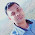# Java, .NET, PHP, PYTHON, ANGULAR, ML, Data Science, Testing, CI Tutorials in Easy Languages.

## "Best Software Training, Internship, Project Development center of Indore India, Helpline 780506-3968"

Image Processing with SciPy – scipy.ndimage:-

scipy.ndimage is a submodule of SciPy which is mostly used for performing an image related operation

It is now deprecated we can not use the misc package on the latest version scipy.

If we want to show image and perform an operation on images then we can use

imageio package to write and read images with the following code.

import imageio
import matplotlib.pyplot as plt
plt.imshow(f)
plt.show()

If we want to flip the images of the actual image using this then we can use flipud() of numpy

```import numpy as np
flip_ud_face = np.flipud(f)
plt.imshow(flip_ud_face)
plt.show()```
```Rotate the image at particular angle in scipy.import numpy as np
from scipy import ndimage, misc

s=ndimage.rotate(f, 45)
plt.imshow(s)
plt.show()
Code to blur image import numpy as np
from scipy import ndimage, misc

#s=ndimage.rotate(f, 45)
s=ndimage.gaussian_filter(f, sigma=3)
plt.imshow(s)
plt.show()http://scipy-lectures.org/advanced/image_processing/```

If you have any doubt in programming or join online classes then you can contact us by comment .

1.# DATA Science ( 7 to 8 PM)

# Matching two Images using Nd-Image(SciPy)

import imageio
import numpy as np
f = input("Name of First Image :-\t")
g = input("Name of Second Image you want to Match :-\t")

import matplotlib.pyplot as plt

plt.imshow(f1)
plt.show()

plt.imshow(g1)
plt.show()

if np.all(f == g):
print("Image is Matched")
else:
print("Image Un-Matched")